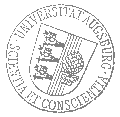# Universität AugsburgInstitut für MathematikAnalysis-Seminar Augsburg-München

Virginie Ehrlacher
CERMICS - Ecole des Ponts ParisTech

spricht am

Donnerstag, 16. Januar 2020

um

17:15 Uhr

im

TUM, Boltzmann-3, Garching, Raum 03.08.011, Etage 3

über das Thema:

## »Moment constrained optimal transport problem: application to quantum chemistry (joint work with A. Alfonsi, R. Coyaud and D. Lombardi)«

 Abstract: The aim of this talk is to present some recent results on a relaxation of multi-marginal Kantorovich optimal transport problems with a view to the design of numerical schemes to approximate the exact optimal transport problem. More precisely, the approximate problem considered in this talk consists in relaxing the marginal constraints into a finite number of moment constraints, while the state space remains unchanged (typically a subset of R^d for some positive integer d). Using Tchakhaloffs theorem, it is possible to prove the existence of minimizers of this relaxed problem and characterize them as discrete measures charging a number of points which scales at most linearly with the number of marginals in the problem. In the particular case of a symmetric multi-marginal problem, like the Coulomb cost optimal transport problem which is the semi-classical limit of the Lévy-Lieb functional , the number of points charged by minimizers is independent of the number of electrons, thus avoiding the curse of dimensionality. This result is strongly linked to the work  and opens the way to the design of new numerical schemes exploiting the structure of these minimizers. Some preliminary numerical results exploiting this structure will be presented.  COTAR, Codina, FRIESECKE, Gero, et KLÜPPELBERG, Claudia. Smoothing of transport plans with fixed marginals and rigorous semiclassical limit of the HohenbergKohn functional. Archive for Rational Mechanics and Analysis, 2018, vol. 228, no 3,, p.891-922.  FRIESECKE, Gero et VÖGLER, Daniela. Breaking the curse of dimension in multi-marginal kantorovich optimal transport on finite state spaces. SIAM Journal on Mathematical Analysis, 2018, vol. 50, no 4, p. 3996-4019.